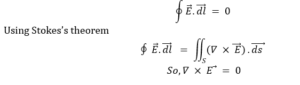Engineering Jobs   »   GATE ELECTRICAL QUIZ, GATE 2022 ELECTRICAL,

# GATE’22 EE: Daily Practices Quiz 20-Aug-2021

GATE 2022 is being organized by IIT, Kharagpur. Through this exam, the qualified candidates get admission into master’s & direct doctoral programs in the field of Engineering/ Architecture/ Technology/ Arts & Science, etc. Qualification in GATE is mandatory for seeking admission to various institutions.

GATE’22 EE: Daily Practices Quiz 20-Aug-2021

Each question carries 2 marks
Negative marking: 2/3 mark

Total Questions: 05
Total marks: 10
Time: 12 min.

Q1. An electrostatic field is said to be conservative when
(a) The divergence of the field is equal to zero.
(b)The curl of the field is equal to zero.
(c) The curl of the field to -∂B/∂t.
(d)The Laplacian of the field is equal to με ∂^2 E)/(∂t^2 ).

Q2. In which of these is reverse recovery time nearly zero?
(a) Zener diode
(b) Tunnel diode
(c) Schottky diode
(d) PIN diode

Q3. The Laplace transform of e^(-2t)sin(2ωt) is
(a) 2s/(s+2)^2+2ω^2
(b) 2ω/(s-2)^2+4ω^2
(c) 2ω/((s+2)^2+4ω^2
(d) 2s/(s-2)^2+2ω^2

Q4. A 10 KVA, 2200/220 V transformer gave the following test results:
Open circuit test:
HV side open; V=220 V, I=1.5 A, W=150 W.
Short-circuit test:
LV side short-circuited; V=115 V, I=rated, W=200 W
The half-full load efficiency of the transformer operating at unity power factor is:
(a) 95.15 %
(b) 95.5 %
(c) 96.15 %
(d) 96.5 %

Q5. A 6-pole, 3-phase alternator running at 1000 rpm supplies to an 8-pole, 3-phase induction motor which has a rotor current of frequency 2 Hz. The speed at which the motor operates is
(a) 750 rpm
(b) 720 rpm
(c) 960 rpm
(d) 1000 rpm

SOLUTIONS

S1. Ans.(b)
Sol. An electrostatic field is said to be conservative when the closed line integral of the field is zeroS2. Ans.(c)
Sol. In Schottky diode there is no charge storage and hence almost zero reverse recovery time.

S3. Ans.(c)
Sol. We know that,
e^(-at) sin bt ↔┴( L.T ) b/(〖(s+a)〗^2+b^2 )
Here,
b = 2ω
a = 2
So, L{e^(-2t)sin(2ωt)} = 2ω/(〖(s+2)〗^2+4ω^2 )

S4. Ans.(c)
Sol. From OC test: W=150 W=P_i
From SC test: W=200 W=P_cu (fl)
Copper loss at half-full load=(〖1/2)〗^2×200=50 W
Iron loss at half-full load=150 W (constant loss)

S5. Ans.(b)
Sol. Supply frequency(f)=(1000×6)/120=50 Hz
Slip frequency: sf=2 (ACQ)
∴slip(s)=2/50=0.04
∴N_s=120f/P=(120×50)/8=750 rpm
∴N_r=N_s (1-s)=750×(1-0.04)=720 rpm

Sharing is caring!

Thank You, Your details have been submitted we will get back to you.